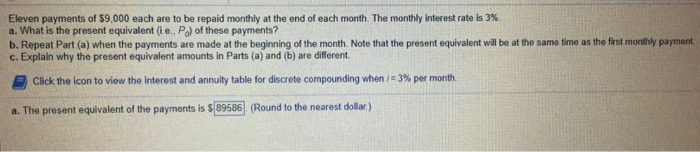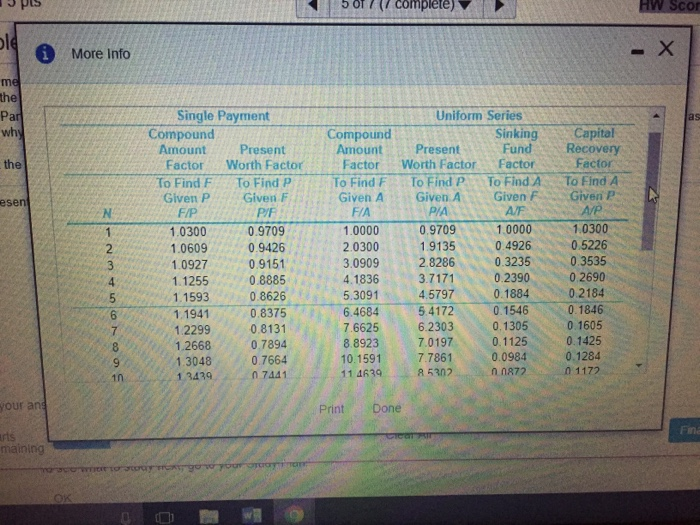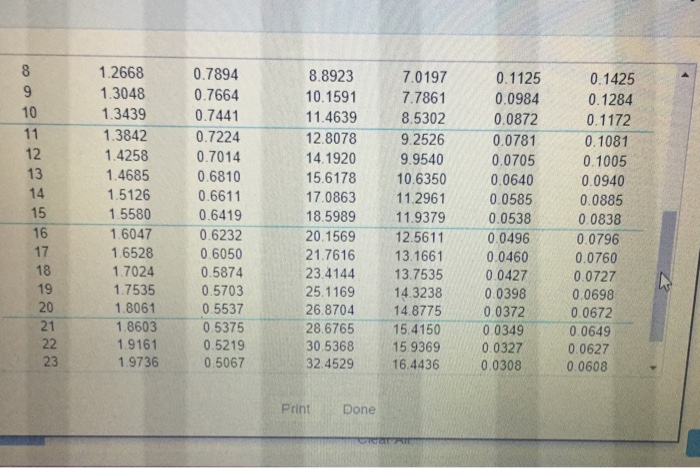# Question & Answer: Engineering economy homework…..

Engineering economy homeworkEleven payments of \$9,000 each are to be repaid monthly at the end of each month The monthly interest rate is 3%. a. What is the present equivalent (ie., Po) of these payments? b. Repeat Part (a) when the payments are made at the beginning of the month. Note that the present equivalent will be at the same time as the first monthly payment c. Explain why the present equivalent amounts in Parts (a) and (b) are different 昌Click the icon to view the interest and annuity table for discrete compounding when : 3% per month. a. The present equivalent of the payments is \$ 89586(Round to the nearest dollar)